Mobile QR Code1. (Dept. of Electronic Engineering, Hanyang University, Seoul 04763, Korea)

## I. INTRODUCTION

In all of the above methods, the timing skew was calibrated using converted digital output of TI-ADCs based on some assumptions about the relationship between the output data and the timing skew. In these methods, because all of the signal and clock paths is in the feedback loop of the skew calibration, the timing skew caused by any of the components can be corrected in principle. However, this universal correction capability comes with a price of complicated calibration algorithms and/or large digital blocks to process them, or the restrictions on the kind of input signals to the TI-ADC (i.e., a significantly smaller bandwidth than the Nyquist bandwidth.)

This paper presents a very simple calibration method, which is based on the observation that a majority of the timing skew can be removed by aligning the sampling clocks for the channel ADC just before they are input to the channel ADCs. At the core of the proposed calibration system are phase-frequency-detector-like timing skew detectors consisting of a pair of D flip-flops. The skew detectors compare the timing of the channel clocks to that of a universal reference clock operating at the aggregate sampling frequency. This is a very simple calibration method that does not require complex calculations in the digital domain. It does not require a large amount of output data to be accumulated for statistical processing either.

In the proposed method, the signal and the clock path after the timing-comparison circuit is not included in the feedback loop for the skew calibration. Therefore, the skew errors originating from the mismatches in those parts cannot be corrected. However, even with the limitation, the proposed method can be attractive because it can remove a bulk of the timing skew with very simple hardware.

This paper is organized as follows. Section II presents the proposed timing skew calibration method. Simulation results are discussed in Section III, and Section IV provides the discussion and conclusion.

## II. PROPOSED FLIP-FLOP BASED TIMING SKEW CALIBRATION METHOD

In this work, we design a skew calibration circuit for a 6-bit 4-channel TI-ADC operating at a sampling frequency of 4 GHz. Fig. 1 shows the block diagram of the 4-channel TI-SAR ADC. An input clock of frequency f$_{\mathrm{s}}$ = 4 GHz is applied to the clock generator, which divides it to generate four channel-clocks of f$_{\mathrm{s}}$/4 = 1 GHz. The channel-clocks are used to sample the input signal for each sub-ADC and to initiate the operation of them. After the four sub-ADCs operate on the input sequentially, the output of the sub-ADCs is combined into a single output stream by a multiplexer (MUX).

In an operation of a TI-ADC, it is very important that the channel clocks are evenly spaced by T = 1/f$_{\mathrm{s}}$ as indicated in Fig. 1. If there is any skew between them, this can significantly degrade the performance of the TI-ADC . Fig. 2 shows the relationship between the size of the timing skew and the SNDR degradation obtained from behavioral simulations of a 4-channel 6-bit TI-ADC assuming sinusoidal inputs. The simulations were performed applying a positive skew of ${\Delta}$T to the second channel and a negative skew of the same size to the third channel. The x- and y-axis represent the relative timing skew (${\Delta}$T/T) and the SNDR reduction, respectively. Results are shown for several input frequencies. Fig. 2 shows that to limit the SNDR reduction from the timing skew at 0.5 dB at the Nyquist input frequency (=f$_{\mathrm{s}}$/2), the skew should be 0.33 % or less. Therefore, we set the target for the skew calibration at 0.3 % relative skew, which corresponds to 750 fs in this work.

##### Fig. 1. Block diagram of a 4-channel TI-ADC.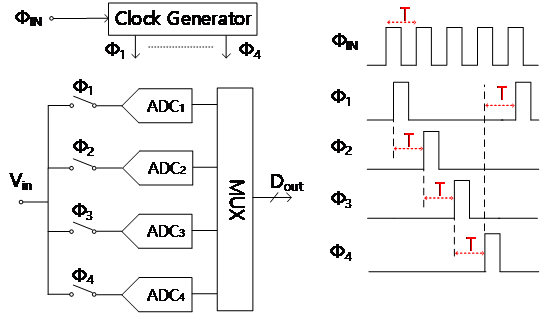##### Fig. 2. Timing skew error vs SNDR degradion for several values of input frequencies.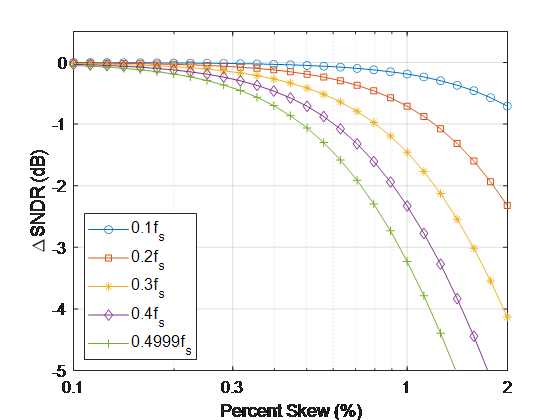### 2. Proposed flip-flop based timing-skew calibration logic

As mentioned in Introduction, in the proposed calibration method, the timing skew between the sampling clocks are removed after comparing the timings of the sampling clocks with that of the reference clock at the input of sub-ADCs. Fig. 3 shows the proposed skew calibration system. Each channel clocks generated by the clock generator is supplied to a sub-ADC after passing through a variable delay line (VDL). A skew detection circuit detects the polarity of the relative timing of a channel clock ${\Phi}$$_{\mathrm{1\sim 4}} to that of the reference clock {\Phi}$$_{\mathrm{REF}}$, which is a copy of the 4-GHz input clock. The digital output of the skew detector is delivered to a counter which controls the delay of the VDL. The timing skew of the channel clocks is not expected to be very large, but it should be fine-tuned. Therefore, VDLs for the channel clocks have 7-bit resolution (binary coded) with step sizes of about 300 fs resulting in total ranges of about 38~ps.

The delay of ${\Phi}$REF itself is controlled by a coarse VDL of which the step-size is much larger than that of the VDLs for the channel clocks. The purpose of the VDL for ${\Phi}$$_{\mathrm{REF}} is to place the timing of {\Phi}$$_{\mathrm{REF}}$ in the middle of the timings of the channel clocks. Whereas the channel clocks are generated by CML clock dividers, the reference clock ${\Phi}$$_{\mathrm{REF}} is produced by a simple chain of inverters. Therefore, depending on the PVT condition, there can be relatively large relative delays between the reference and the channel clocks, which should be compensated by a VDL. The resolution and the step size of the coarse VDL are 3 bits and 30 ps, respectively, resulting in the total coverage of about 200 ps, which is nearly one clock period of the target TI-ADC. We call the VDL for {\Phi}$$_{\mathrm{REF}}$ functions a coarse VDL and those for channel clocks find VDLs.

The calibration logic flow is shown in Fig. 4. The 7-bit digital codes controlling the channel clock VDLs are updated in the direction determined by the skew-polarity detectors which determine the polarity of the skew of each channel clock against ${\Phi}$$_{\mathrm{REF}}. A code reaching an end (i.e., 0000000(2) or 1111111(2)) means that the corresponding channel clock VDL is out of range. If any of the four channel-clock VDLs reaches an end, the delay of the reference clock VDL is changed by one step, and the skew calibration for each channel is repeated with the new coarse delay. Note that step sizes of the reference clock VDL is much larger than the step size of the channel clock VDLs. The calibration method proposed in this paper relies on our ability to determine the relative timings of the channel clocks to that of {\Phi}$$_{\mathrm{REF}}$. Therefore, the design of skew detectors is at the core of our work. In this work, we used very simple skew-polarity detectors consisting of mainly two D-flip flops (DFFs). The schematic of the detector is shown in Fig. 5. Note that while Fig. 5 shows the signal connections of the detector which compares the timing of the second channel clock ${\Phi}$$_{2} with that of {\Phi}$$_{\mathrm{REF}}$, similar detectors exist for other channels.

In Fig. 5, ${\Phi}$$_{\mathrm{REF}} or {\Phi}$$_{2}$ are applied to the clock inputs of the DFFs, and HI is applied to D inputs of both DFFs. The output of the DFF to which the clock arrives earlier will become HI first. This HI output resets the opposite DFF and prevents it from becoming HI when a clock arrives at the DFF later. Therefore, the two interconnected flip-flops store the polarity of the timing skew. For example, if a$_{2}$ = LO and b$_{2}$ = HI, it means that ${\Phi}$$_{2} is ahead of {\Phi}$$_{\mathrm{REF}}$. On the other hand, if a$_{2}$ = HI and b$_{2}$ = LO, it indicates the opposite case. The timing skew must be re-evaluated regularly. To prepare the detector for a new acquisition, the DFFs are reset when ${\Phi}$$_{1}= HI, which is before the arrival of a new {\Phi}$$_{2}$. When ${\Phi}$$_{1} becomes LO again, the reset input of DFFs are connected to the output of the opposite DFF again and the skew detector is ready to accept new clock inputs. The output of the skew detector is accumulated by a counter, of which the output controls a channel clock VDL. Fig. 6 shows the waveform obtained from spice-level simulations using Spectre. We observe very clean waveforms. Although x_{2} rises a little when {\Phi}$$_{\mathrm{REF}}$ arrives, it is soon reset by y$_{2}$. y$_{2}$ itself is reset by CK1.

When the skew is very small, it is possible that neither a$_{2}$ nor b$_{2}$ can fully rise to HI because of their mutual reset operations. If that happens, it can lead to malfunction of the calibration logic. To prevent this, a ‘forced decision’ circuit is used. If none of the output of both DFFs becomes HI by the time ${\Phi}$$_{3} arrives, the FD flag is set, which forces a_{2}= HI and b_{2}= LO until next {\Phi}$$_{1}$arrives.

The input range in which this forced decision occurs corresponds to a kind of dead-zone'', which can limit the ultimate resolution of the skew detection. The width of the dead-zone was estimated to be about 60 fs using simulations. Because it is very small, the forced decision should not affect the calibration performance.

Fig. 7 shows the waveforms corresponding to this ‘forced decision’. Because of the proximity of the arrival of ${\Phi}$$_{\mathrm{REF}} and {\Phi}$$_{2}$, x2 and y2 reset each other resulting in none of them reaching HI by the time CK3 arrives. Then, FD becomes HI and a2 = HI and b2 = LO are produced.

Fig. 8 illustrates an example of the time evolution of the output of the skew-polarity detector and the output of the counter D$_{2}$<6:0> applied to the channel clock VDL which adjusts the timing of the channel clock ${\Phi}$$_{2}. Fig. 8(a) represents the signal evolution before the calibration is completed. In this case, {\Phi}$$_{2}$is leading ${\Phi}$$_{\mathrm{REF}}. Therefore, a_{2}= LO and b_{2}= HI are produced after each detection, which leads to a gradual increase of D_{2}<6:0>, which, in turn, reduces the timing difference between {\Phi}$$_{2}$ and ${\Phi}$$_{\mathrm{REF}}. Fig. 8(b) shows the signals after the calibration is completed. At this time, the a_{2} and b_{2} toggles between HI and LO. An example of the time evolution of the digital codes controlling {\Phi}$$_{\mathrm{REF}}$ and ${\Phi}$$_{2} is illustrated in Fig. 9. It shows what happens when the counter for a channel clock reaches an end during skew calibration. Here, at the beginning {\Phi}$$_{2}$leads ${\Phi}$$_{\mathrm{REF}} and the code for {\Phi}$$_{2}$is gradually increased until it reaches 1111111$_{\mathrm{(2)}}$. Then, the code for ${\Phi}$REF is reduced by one bit. In this particular example, the reduction by one bit of the code for ${\Phi}$REF is enough to make ${\Phi}$$_{\mathrm{REF}} leads {\Phi}$$_{2}$. Therefore, the code for ${\Phi}$$_{2}now begins to come down until it toggles between two values. If a one-bit reduction of the code is not enough to make {\Phi}$$_{\mathrm{REF}}$ lead ${\Phi}$$_{2}, then {\Phi}$$_{\mathrm{REF}}$ is reduced repeatedly until it leads ${\Phi}$$_{2}. If there is a mismatch between the clock-to-Q delays of the two DFFs in a skew detection circuit, the mismatch cannot be corrected and will show up as the timing skew of the channel clock. We performed Monte Carlo simulations to measure the distribution of the mismatch of the clock-to-Q delays of DFFs used in our design. The results are shown in Fig. 10, where the x-axis represents the timing difference between {\Phi}$$_{\mathrm{REF}}$ and ${\Phi}$$_{2}, and the y-axis represents the probability of an UP signal (i.e., the probability that {\Phi}$$_{2}$ is determined to have arrived earlier than ${\Phi}$$_{\mathrm{REF}}). We performed 100 iterations for each time difference. From Fig. 10, we estimate that the 1-sigma value of the residual skew from the clock-to-Q mismatch is about 600 fs. Fig. 2 predicts that this would result in an SNR reduction of about 0.26 dB for a sinusoidal input at the Nyquist frequency. The mismatch between the threshold voltages (V_{\mathrm{th}}) of the sampling transistors can contribute to effective timing jitter. The results of the Monte-Carlo simulations indicate that the V_{\mathrm{th}} mismatch is about 5 mV. This, when combined with a 1/20-V/ps-slope of the sampling clock edge, produces jitter of about 100 fs. Therefore, we expect that his should not degrade the jitter performance of 4-GS/s TI-ADCs. Finally, the mismatches between routings for different channels are not calibrated by this method and contribute to the residual skew. The mismatches include following: i) those between the reference clock paths from the reference clock generator to D-FF comparators, ii) those between the channel clock paths from D-FF comparators to the sampling clock input of channel ADCs, iii) those between the signal paths to channel ADC inputs. To estimate the skew from those mismatches, we performed post-layout simulations while changing the length of the path. From the simulations, we found that even with a very large path-length difference of 10 {\mu}m, the delay variation was less than 50 fs only. Therefore, we expect that the path-length mismatch should not degrade the jitter performance of the envisioned TI-ADCs too much. ##### Fig. 3. Block diagram of the proposed skew calibration system.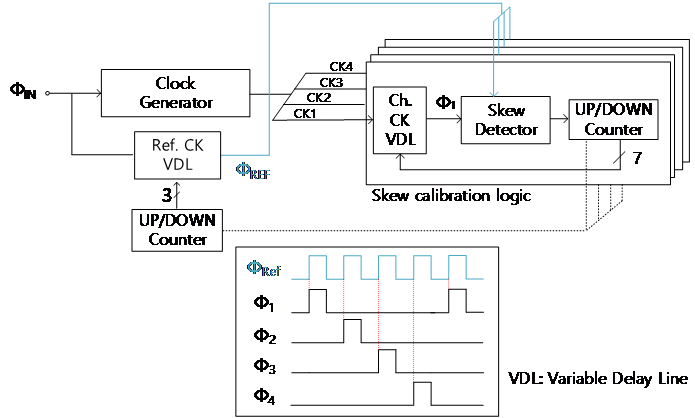##### Fig. 4. Proposed calibration logic flow.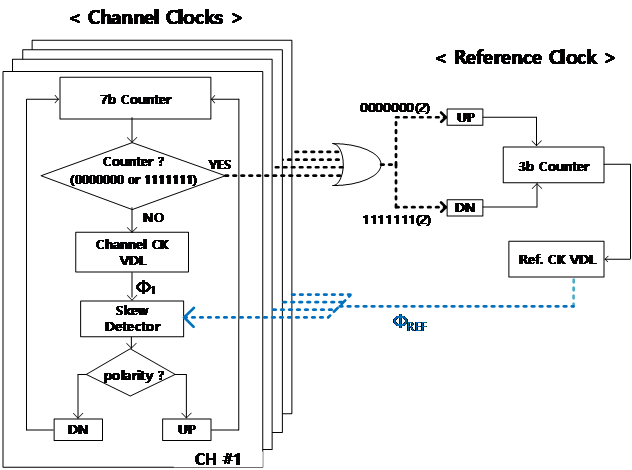##### Fig. 5. Proposed timing skew polarity detection circuit.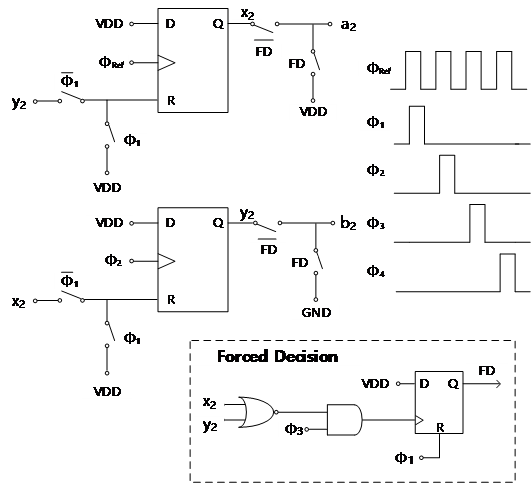##### Fig. 6. Waveforms showing normal timing-skew polarity detection operation of CK2. (see to Fig. 5).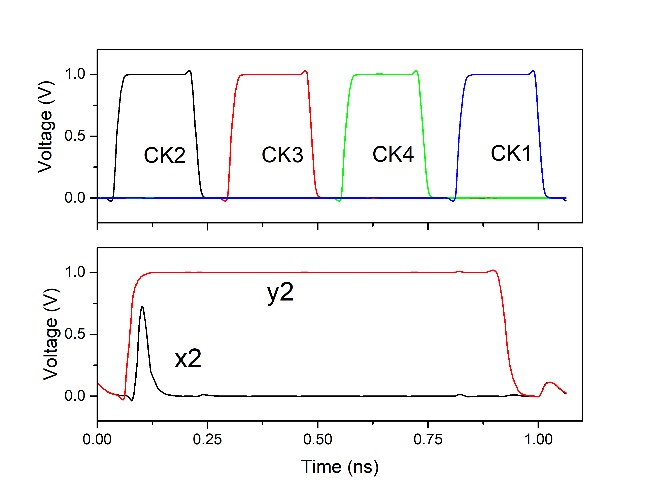##### Fig. 7. Waveforms corresponding to forced decision'' operation of CK2 (see Fig. 5).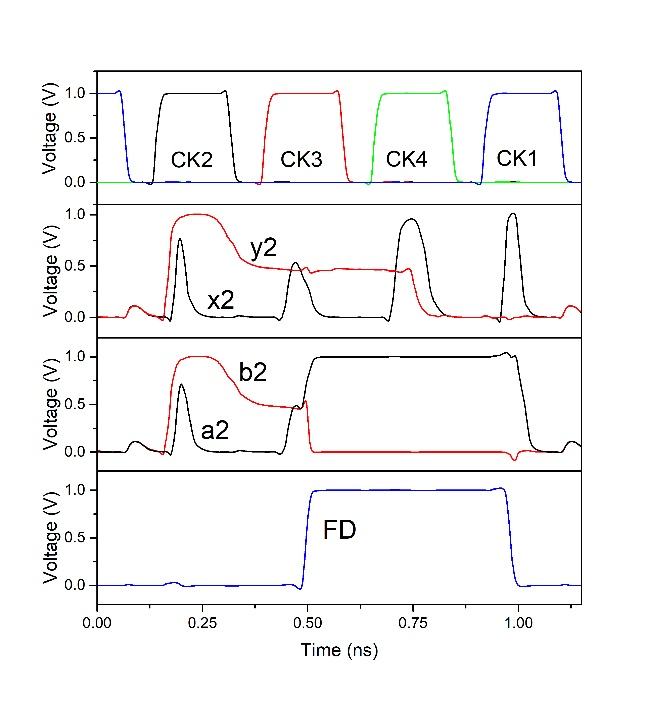##### Fig. 8. Calibration logic output with digital code of delay line. D_{2} is the digital code for the VDL for the 2^{\mathrm{nd}} channel: (a) being calibrated; (b) after successful calibration.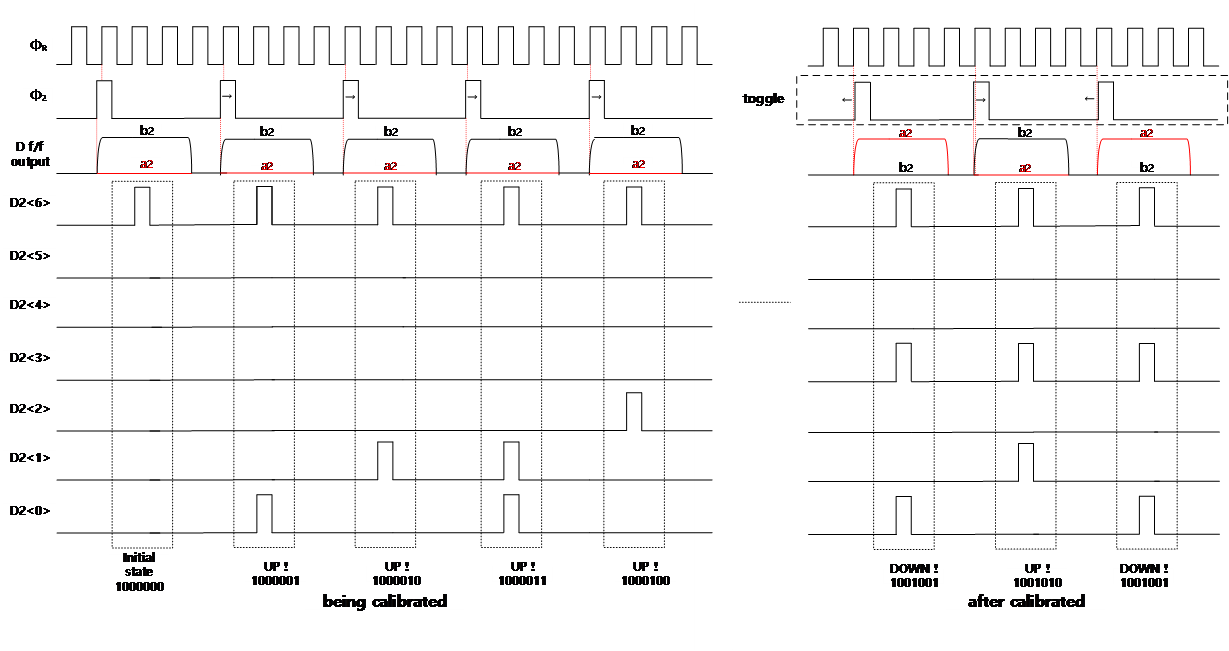##### Fig. 9. Movement of each channel clock and {\Phi}$$_{\mathrm{REF}}$.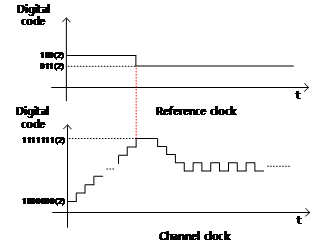##### Fig. 10. Sensitivity to mismatch of two flip flops.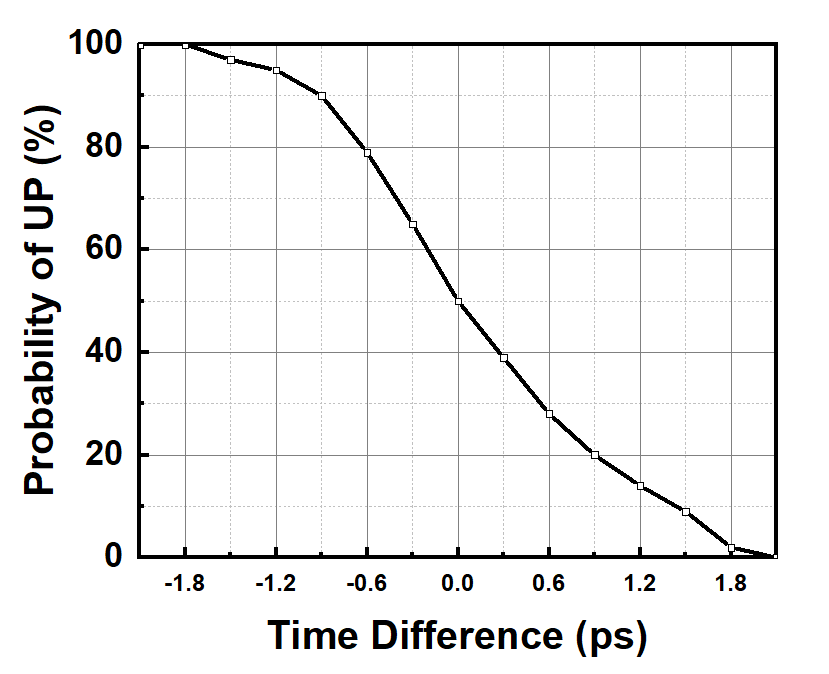### 3. Circuit Implementation

Fig. 11 shows a block diagram of the clock generation circuit used in this work which produces four channel clocks and the reference clock ${\Phi}$$_{\mathrm{REF}}. The channel clocks are produced by a current-mode logic CML frequency divider, of which the structure is similar to that of . The timing of the channel clocks are controlled by shunt-capacitor-inverter (SCI) VDLs. In SCI VDLs, the delay is controlled by switching the bottom-plate connections of the capacitors loading inverters. The resolution of the skew calibration is limited by the step-size of the variable delay of VDLs. In II-1, it was observed that step-size of 800 fs was needed to limit the SNR reduction to below 0.5 dB. In this work, step-sizes of about 300 fs were used to secure margins. The delay of the reference clock is controlled by a variable-path delay line which is suitable for a pico-second-range resolution. When one of the VDL delays for the channel clocks reaches an end, the delay for {\Phi}$$_{\mathrm{REF}}$ should be changed. Then, with the new ${\Phi}$$_{\mathrm{REF}}$ delay, the channel clock VDL delays are adjusted again as already illustrated in Fig. 9. When the delays of a channel clock VDL and the reference clock VDL are changed simultaneously, there is a risk that the delays interfere with each other generating oscillation at the worst case. Especially in our circuit, the ‘end of the range’ signal is generated by a sub channel circuit operating at f$_{\mathrm{s}}$/4 and kept HI for three reference clock periods (=3T), while the reference clock VDL is adjusted every reference clock period. Therefore, if the ‘end of the range’ signal is directly used for the reference clock VDL adjustment, the VDL is adjusted in consecutive three steps producing large transient perturbation which leads to oscillation.

Fig. 12 shows the circuit to prevent this and its timing diagram of operation. In Fig. 12, ER_U (or ER_L) represents the signal indicating that the counter for a channel clock VDL reaches the upper (or lower) end. UP (or DN) is the signal input to the counter for the coarse VDL to indicate that a 1-bit increase (or decrease) of the control bits Q<2:0> for the coarse VDL is required. We can observe in the timing diagram in Fig. 12, even though the ER_U signal has length of 3T, this circuit reduces the length of UP (or DN) signal to one clock cycle. Therefore, for a single ‘end of range event’ the reference clock counter is activated only once.

##### Fig. 11. Block diagram of the clock generation system.##### Fig. 12. Proposed structure to prevent undesired interactions between delays for channel clocks and the reference clock.## III. SIMULATION RESULTS

The operation of the proposed timing skew calibration circuit was verified by SPICE level simulations including post-layout simulations after implementing it with a SAMSUNG 28-nm CMOS process. Fig. 13 shows the layout of the calibration circuit occupying 176.3x79.2 mm$^{2}$. Note that de-coupling caps have been eliminated from the figure for the sake of clarity. The highlighted Y-shaped structure in the upper half represents the routing path of the reference clock to skew-detection circuits, and the highlighted V-shaped structure at the bottom represents the routing of the channel clocks from the CML divider to CML to CMOS converter.

To verify the operation of the proposed timing-skew calibration circuit, we performed Monte-Carlo simulations including the mismatch and process variations (100 iterations). The sampling frequency was 4 GHz (4x1 GHz). The residue skew values obtained from the simulations are shown in Table 1. Ideally, the delay between CK$_{\mathrm{K+1}}$ and CK$_{\mathrm{K}}$ should be 1/f$_{\mathrm{s}}$ ${\approx}$ 250 ps. The values listed in Table 1 represent the skew of the delay from this ideal value. Before Cal.'' represents the timing skew of the four output clocks of the CML divider, and After Cal.'' represents the skew measured after the clocks go through the calibration circuit. We observe that the skew of about 4.0 ps before calibration is reduced to about 900 fs after calibration. According to the behavioral simulation results in Fig. 2, a skew of 4.0 ps and 900 fs result in 5.8 dB and 0.6 dB reduction of the SNDR, respectively, with the sinusoidal input at Nyquist frequency. Therefore, we can say that the proposed timing skew calibration method enables a large performance enhancement.

The post-layout simulation results of the calibration logic are shown in Table 2. The parasitic extraction was carried out based on the layout of Fig. 13. The same conditions were used as those for Table 1. Before the calibration, we can observe relatively large skews from the layout mismatch. However, after calibration, we can observe that timing skew error is greatly reduced.

##### Fig. 13. Layout of the proposed calibration circuit.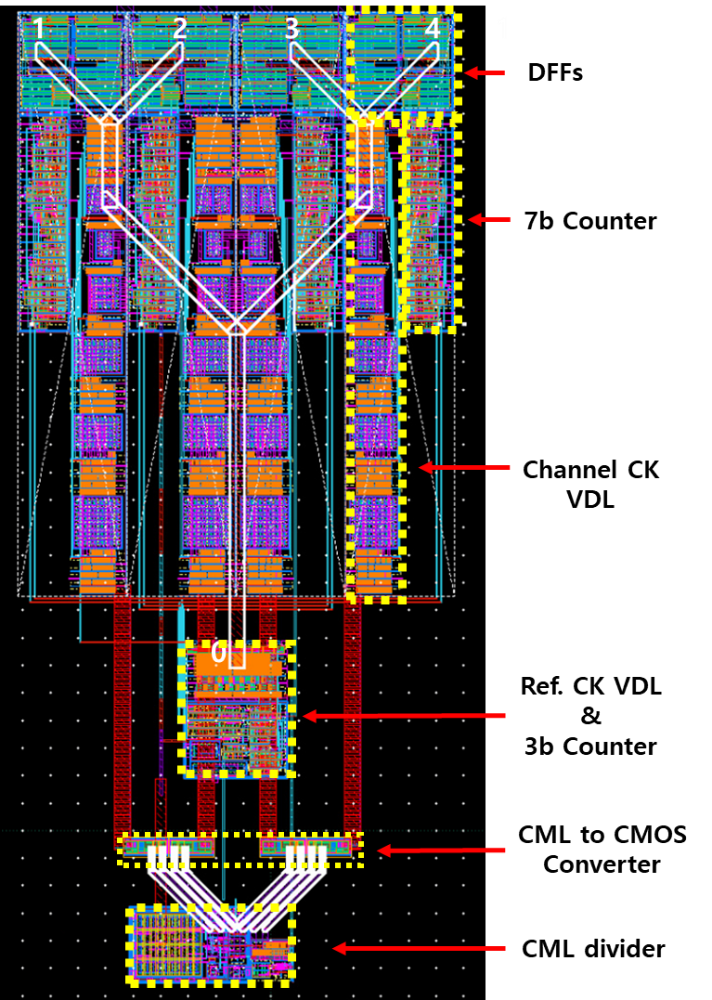##### Table 1. Monte-Carlo simulation results (100 samples)
 Before Cal. After Cal. (Std Dev) Ch 1 ~ Ch 2 4.079 ps 0.947 ps Ch 2 ~ Ch 3 3.720 ps 0.917 ps Ch 3 ~ Ch 4 4.048 ps 0.952 ps Ch 4 ~ Ch 1 4.285 ps 0.840 ps
##### Table 2. Post layout simulation results
 Before Calibration After Calibration Ch 1 ~ Ch 2 + 10.65 ps - 0.178 ps Ch 2 ~ Ch 3 + 3.68 ps + 0.063 ps Ch 3 ~ Ch 4 - 4.51 ps - 0.583 ps Ch 4 ~ Ch 1 - 2.21 ps - 0.153 ps

## IV. DISCUSSIONS AND CONCLUSIONS

This brief presented a timing skew calibration method for TI-ADCs. A new simple and effective method to calibrate the timing skew error was proposed and the proper operation of the calibration circuit based on the method was verified by SPICE level simulations.

The fundamental disadvantage of the proposed method to other calibration methods using final digital output of TI-ADC is that the proposed method cannot correct the skew caused by the mismatch in the layout of the routing between the input to the skew detection circuit and the clock input of the ADC. This is because the calibration is performed by matching the timing of the clocks at the input of the skew detection circuit. It cannot remove the skew caused by the mismatch between the input signal lines to ADCs, either. However, the advantage of this method is that it needs very simple circuitry centered on the proposed skew detection circuits, each of which mainly consisting of two DFFs. It does not need complicated digital calculations or a large digital logic block. Therefore, despite its disadvantage, we believe the proposed skew calibration circuit can find its use where a simple calibration circuit is desired.

## ACKNOWLEDGMENTS

This work was supported by the Korea Institute for Advancement of Technology (KIAT) grant funded by the Korea Government (MOTIE) (P0017011, HRD Program for Industrial Innovation). This work was also supported by National R&D Program through the National Research Foundation of Korea (NRF) funded by Ministry of Science and ICT (No. 2020M3H2A1076786). The CAD tools were provided by IC Design Center (IDEC), Korea.

## References

1
J. Son and Y. Jang, “A 10-bit 10-MS/s Asynchronous SAR ADC with Input Offset Calibration using Capacitor DAC,” J. Semiconductor Technology and Science, Vol. 20, No. 6, pp. 518-525, Dec. 2020.2
I. Yeo and B. Lee, “A 12-bit 40 MS/s SAR ADC with Digital Foreground Self-calibration for Capacitor Mismatches,” J. Semiconductor Technology and Science, Vol. 20, No. 01, pp. 105-118, Feb. 2020.3
L. Kull et al., “A 3.1 mW 8b 1.2 GS/s Single-Channel Asynchronous SAR ADC With Alternate Comparators for Enhanced Speed in 32 nm Digital SOI CMOS,” in IEEE Journal of Solid-State Circuits, vol. 48, no. 12, pp. 3049-3058, Dec. 2013, doi: 10.1109/JSSC.2013.2279571.4
M. El-Chammas and B. Murmann, “General Analysis on the Impact of Phase-Skew in Time-Interleaved ADCs,” in IEEE Transactions on Circuits and Systems I: Regular Papers, vol. 56, no. 5, pp. 902-910, May 2009, doi: 10.1109/TCSI.2009. 2015206.5
S. Lee, A. P. Chandrakasan and H. Lee, “22.4 A 1GS/s 10b 18.9mW time-interleaved SAR ADC with background timing-skew calibration,” 2014 IEEE International Solid-State Circuits Conference Digest of Technical Papers (ISSCC), 2014, pp. 384-385, doi: 10.1109/ISSCC.2014.6757480.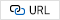6
M. El-Chammas and B. Murmann, “A 12-GS/s 81-mW 5-bit Time-Interleaved Flash ADC With Background Timing Skew Calibration,” in IEEE Journal of Solid-State Circuits, vol. 46, no. 4, pp. 838-847, April 2011, doi: 10.1109/JSSC.2011.2108125.7
H. -W. Kang, H. -K. Hong, S. Park, K. -J. Kim, K. -H. Ahn and S. -T. Ryu, “A Sign-Equality-Based Background Timing-Mismatch Calibration Algorithm for Time-Interleaved ADCs,” in IEEE Transactions on Circuits and Systems II: Express Briefs, vol. 63, no. 6, pp. 518-522, June 2016, doi: 10.1109/TCSII.2016.2530819.8
M. Guo, J. Mao, S. -W. Sin, H. Wei and R. P. Martins, “A 29mW 5GS/s Time-interleaved SAR ADC achieving 48.5dB SNDR With Fully-Digital Timing-Skew Calibration Based on Digital-Mixing,” 2019 Symposium on VLSI Circuits, 2019, pp. C76-C77, doi: 10.23919/VLSIC.2019.8778077.9
J. Song, K. Ragab, X. Tang and N. Sun, “A 10-b 800-MS/s Time-Interleaved SAR ADC With Fast Variance-Based Timing-Skew Calibration,” in IEEE Journal of Solid-State Circuits, vol. 52, no. 10, pp. 2563-2575, Oct. 2017, doi: 10.1109/JSSC.2017.2713523.10
J. Liu, C. Chan, S. Sin, U. Seng-Pan and R. P. Martins, “Accuracy-Enhanced Variance-Based Time-Skew Calibration Using SAR as Window Detector,” in IEEE Transactions on Very Large Scale Integration (VLSI) Systems, vol. 27, no. 2, pp. 481-485, Feb. 2019, doi: 10.1109/TVLSI.2018. 2874772.11
R. Nonis, E. Palumbo, P. Palestri and L. Selmi, “A Design Methodology for MOS Current-Mode Logic Frequency Dividers,” in IEEE Transactions on Circuits and Systems I: Regular Papers, vol. 54, no. 2, pp. 245-254, Feb. 2007, doi: 10.1109/TCSI.2006.885999.Ji-Hun Lim received the B.S. degree in Electronics Engineering from Seokyeong University, Seoul, Korea, in 2020. He was with Hanyang University for his M.S. degree in Electronics Engineering. He is now with Samsung Electronics, Hwasung Korea. His research focuses on analog/mixed-signal circuit design including data converters and wireline transceivers.

Sang-Gyu Park received B.S. and M.S. degrees in Electronics Engineering from Seoul National University in 1990 and 1992, respectively and received Ph.D. degree in Electrical and Computer Engineering from Purdue University in 1998. He worked at AT&T Laboratories-Research from 1998 to 2000 and joined the faculty of Hanyang University in 2000, where he is a professor in Electronics and Computer Engineering. His research area is the mixed-signal CMOS circuit design, with focus on delta-sigma oversampling data converters, high speed SAR ADCs and memory interface circuits.